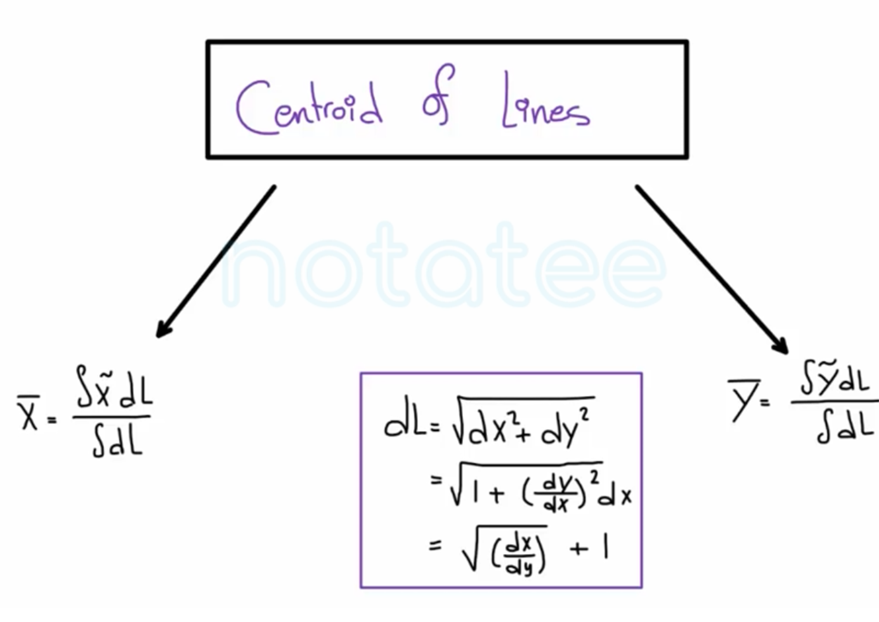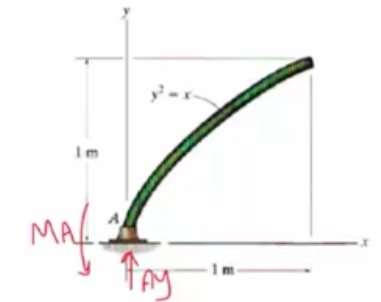Need Help?

Subscribe to Statics

###### \${selected_topic_name}
• NotesThe uniform rod is bent into the shape of a parabola  and has a weight per unit length of  $100 \mathrm{N} / \mathrm{m}$  . Determine the  reactions at the fixed support  $A$$d L=\sqrt{d x^{2}+d y^{2}}$

$=\sqrt{\left(\frac{d x^{2}}{d y^{2}}\right)+1} \ d y$

$=\sqrt{\left(\frac{d x}{d y}\right)^{2}+1} \ d y$

$\left|\begin{array}{c}{y^{2}=x} \\ {2 y d y=d x} \\ {\frac{d x}{d y}=2 y} \\ {\left(\frac{d x}{d y}\right)^{2}=4 y^{2}}\end{array}\right.$

$L=\int_{0}^{1} \sqrt{1+4 y^{2}} d y$

$L=\int_{0}^{1} d l=1.48 m$

$\overline{x}=\frac{\int \tilde{x} d l}{\int d l} =\frac{\int_{0}^{1} y^{2} \sqrt{1+4 y^{2}} d y}{1.48}= \frac{0.606}{1.48}=0.41 \mathrm{m}$

$+\uparrow \sum F_ y=0 \quad\longrightarrow A_ y-1.48(100) =0 \longrightarrow A_ y=148 N \uparrow$

$\sum M_ A=0 \quad\longrightarrow M_ A-(148)(0.41)=M_{A}=60.6 \mathrm{N}.{m}$